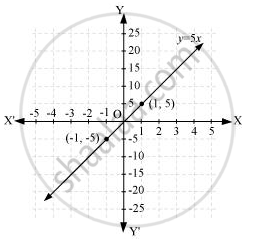# If the work done by a body on application of a constant force is directly proportional to the distance travelled by the body, express this in the form of an equation in two variables and draw the graph of the same by taking the constant force as 5 units - Mathematics

If the work done by a body on application of a constant force is directly proportional to the distance travelled by the body, express this in the form of an equation in two variables and draw the graph of the same by taking the constant force as 5 units. Also read from the graph the work done when the distance travelled by the body is

(i) 2 units (ii) 0 unit

#### Solution

Let the distance travelled and the work done by the body be x and y respectively.

Work done ∝ distance travelled

y ∝ x

y = kx

Where, k is a constant

If constant force is 5 units, then work done y = 5x

It can be observed that point (1, 5) and (−1, −5) satisfy the above equation. Therefore, these are the solutions of this equation. The graph of this equation is constructed as follows.(i)From the graphs, it can be observed that the value of corresponding to x = 2 is 10. This implies that the work done by the body is 10 units when the distance travelled by it is 2 units.

(ii) From the graphs, it can be observed that the value of corresponding to x = 0 is 0. This implies that the work done by the body is 0 units when the distance travelled by it is 0 unit.

Concept: Graph of a Linear Equation in Two Variables
Is there an error in this question or solution?
Chapter 4: Linear Equations in two Variables - Exercise 4.3 [Page 74]

#### APPEARS IN

NCERT Class 9 Maths
Chapter 4 Linear Equations in two Variables
Exercise 4.3 | Q 6 | Page 74

Share